Next: Implementation Up: Newton method for solving Previous: Newton method for solving   Contents

Mathematical background

Let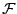be a system ofequations in theunknowns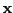and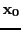be an estimate of the solution of the system. Let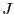be the Jacobian matrix of the system of equation. Then the iterative scheme defined by: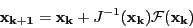(2.6)

starting withmay converge toward a solution of the system.

A simplified Newton method consist in using a constant matrix in the classical Newton method, for example the inverse Jacobian matrix at some point like. The iterative scheme become: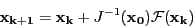(2.7)

Although the simplified method may need a larger number of iteration before converging than the classical scheme each iteration has a lower computation time as there is no computation of the inverse of the Jacobian matrix. This method may also encounter convergence problem as it has a convergence ball smaller than the classical Newton method.

Newton method has advantages and drawbacks that need to be known in order to use it in the best way:

• it may really be fast: this may be important, for example in real-time control
• it is very simple to use
• but it does not necessarily converge toward the solution "closest" to the estimate (see the example in section 15.1.2)
• but it may not converge. Kantorovitch theorem (see section 3.1.2) enable to determine the size of the convergence ball but this size is usually small (but quite often in practice the size is greater than the size given by the theorem which however is exact in some cases)
• but a numerical implementation of Newton may overflowNext: Implementation Up: Newton method for solving Previous: Newton method for solving   Contents
Jean-Pierre Merlet 2012-12-20